﻿ Confidence Interval

# Confidence Interval

This appendix describes the method of confidence interval computation for a one-dimensional normal distribution.

## Cumulative Probability

The cumulative probability is the likelihood that the value of a random variable is within a specific range.

$P\left( a \leq X \leq b \right)$

Let us return to the pizza delivery distribution example (see Essential background I section). We want to find the likelihood that the pizza in city 'A' would be delivered within 33 minutes:

$P\left( 0 \leq X \leq 33 \right)$

Reminder, the pizza delivery time in the city 'A' is normally distributed with a mean of 30 minutes and a standard deviation of 5 minutes $$\left( \mu=30, \sigma=5 \right)$$.

We need to find the area under the PDF curve between zero and 33 minutes: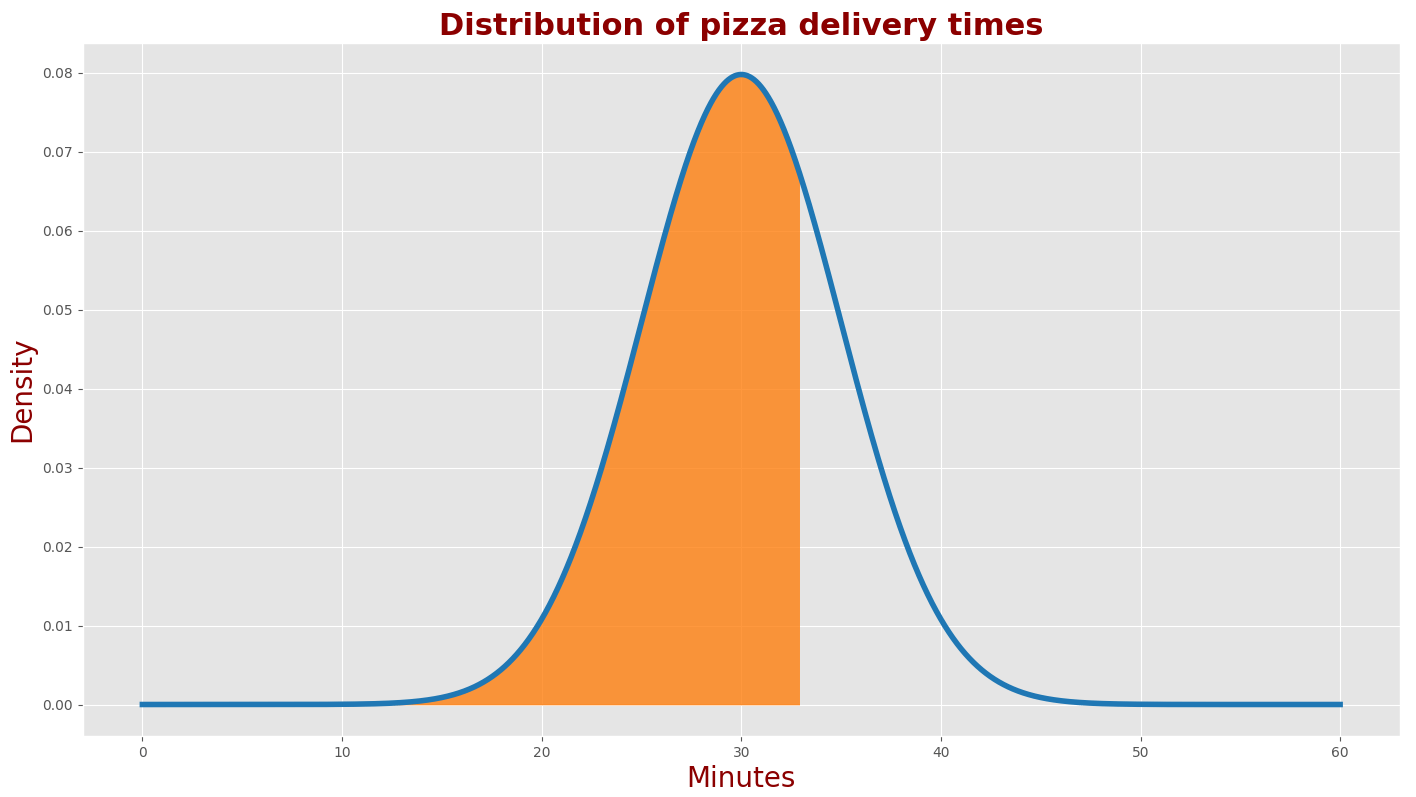The filled area under Gaussian is given by:

$F \left( x;\mu,\sigma^{2} \right) = \frac{1}{\sqrt{2\pi\sigma^{2}}} \int_{0}^{33}exp \left(\frac{-(x-\mu)^2}{2\sigma^{2}} \right)dx$

Don't worry. We won't need to compute this integral.

Let us define a standardized score (also called a z-score) to simplify the problem.

z-score is a standardized random variable with a mean of 0 and a standard deviation of 1 $$\left( \mu=0, \sigma=1 \right)$$.

$z = \frac{x-\mu}{\sigma}$

A z-score defines the distance of $$x$$ from the mean in units of standard deviations. For example:

• If $$z-score=1$$, the value of $$z$$ is one standard deviation above the mean.
• If $$z-score=-2.5$$, the value of $$z$$ is 2.5 standard deviations below the mean.
• If $$z-score=0$$, the value of $$z$$ equals the mean.

The pizza delivery time in city 'A' is a random variable with a mean of 30 and a standard deviation of 5 $$\left( \mu=30, \sigma=5 \right)$$.

z-score for 33 minutes is:

$z = \frac{33-30}{5}=0.6$

z-score for 0 minutes is:

$z = \frac{0-30}{5}=-6$

The PDF of $$z$$ is a standard normal distribution:

$F \left( z \right) = \frac{1}{\sqrt{2\pi}}exp \left(-0.5z^{2} \right)$

The cumulative probability is the area under the PDF between $$-\infty$$ and $$z$$.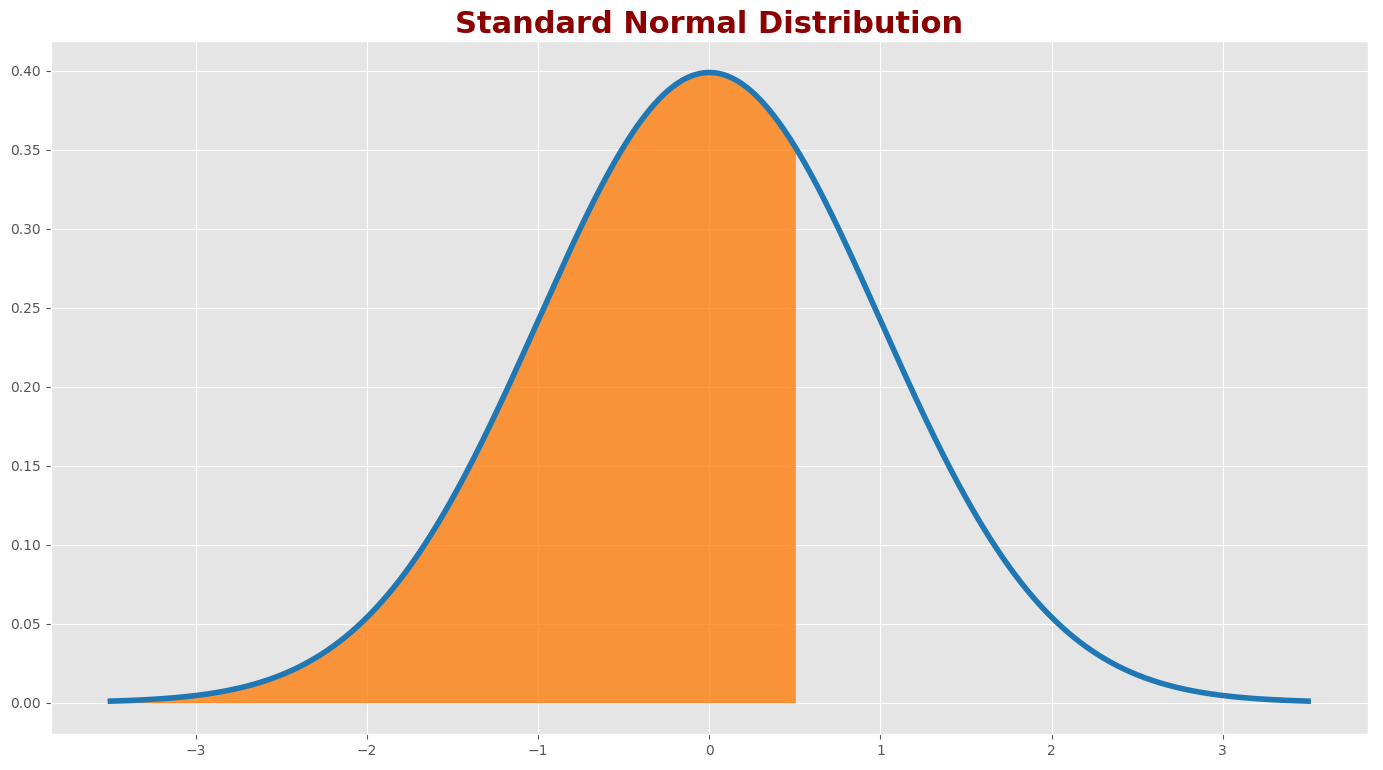The Cumulative Probability of $$z$$ is given by:

$CP \left( z \right) = \frac{1}{\sqrt{2\pi}} \int_{-\infty}^{z}exp \left( -0.5z^{2} \right)dz$

For our example, we need to find the following:

$P(-6 \leq z \leq 0.6)= CP(z=0.6)- CP(z=-6)$

Calculating the PDF integral is not straightforward and requires much work. The faster method is to use statistical z-score tables or computer software packages.

z-score tables contain cumulative probabilities for different z-scores. The following figure exemplifies the location of the cumulative probability for z-score (z=0.6).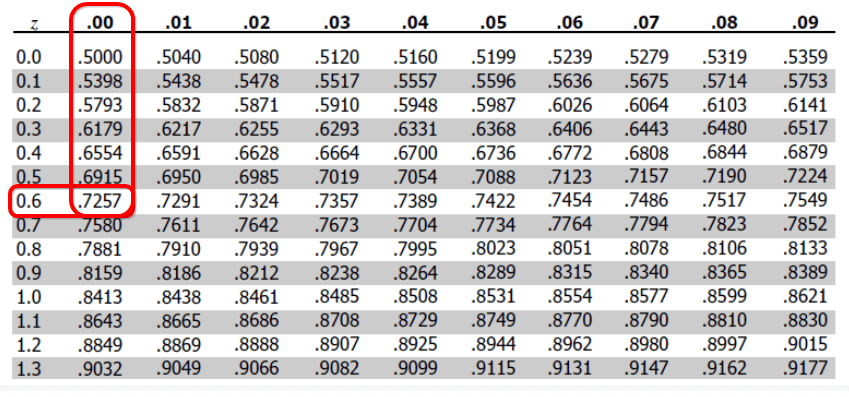$CP \left( z = 0.6 \right) = 0.7257$

You can use scientific computer software packages for a z-score integral computation.

The following commands compute the z-score integral in different computer software packages:

Computer Software Package Command
Python  from scipy.stats import norm 
 norm.cdf(z) 
MATLAB  normcdf(z) 
Excel  NORM.DIST(z, 0, 1, TRUE) 

from scipy.stats import norm

norm.cdf(0.6)
0.7257468822499265

norm.cdf(-6)
9.865876450376946e-10


normcdf(0.6)
0.7257

normcdf(-6)
9.8659e-10


$P(-6 \leq z \leq 0.6) = 0.7257-0 = 0.7257$

The likelihood of having a pizza in city 'A' within 33 minutes is 72.57%.

Or in other words, the pizza delivery time 72.57 percentile in city 'A' is 33 minutes.

Hint: When using computer software packages, you don't need to calculate the z-score. You can specify the mean and standard deviation as an argument of the software function.

The following commands compute the cumulative distribution in different computer software packages:

Computer Software Package Command
Python  from scipy.stats import norm 
 norm.cdf(x, mu, sigma) 
MATLAB  norm.cdf(x, mu, sigma) 
Excel  NORM.DIST(x, mu, sigma, TRUE) 

from scipy.stats import norm

norm.cdf(33, 30, 5)
0.7257468822499265


normcdf(33, 30, 5)

0.7257


## Normal inverse cumulative distribution

In this chapter, we would like to answer a reverse question. What is the cumulative distribution for a given percentile?

For example, what is the 80th percentile for the pizza delivery time in the city' A'?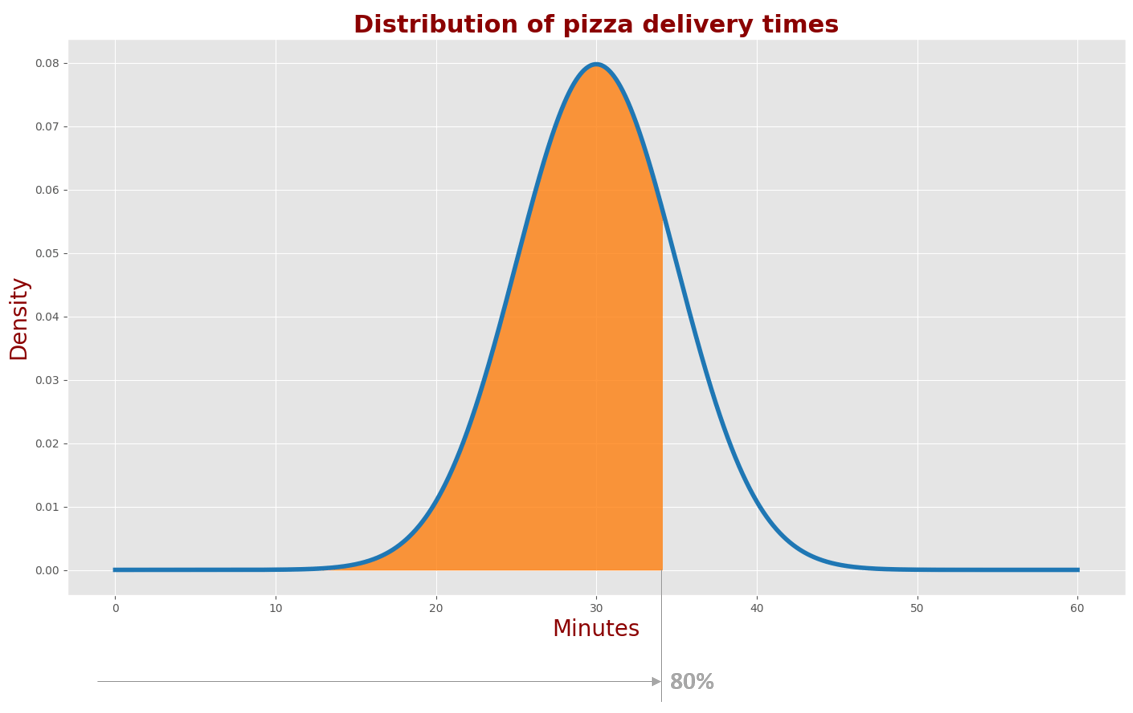One method is to use the z – score table:

• In the table below, find the cumulative distribution value closest to 0.8.
• The $$z-score$$ is a combination of the row $$z-value$$ and column $$z-value$$: $$z=0.84$$.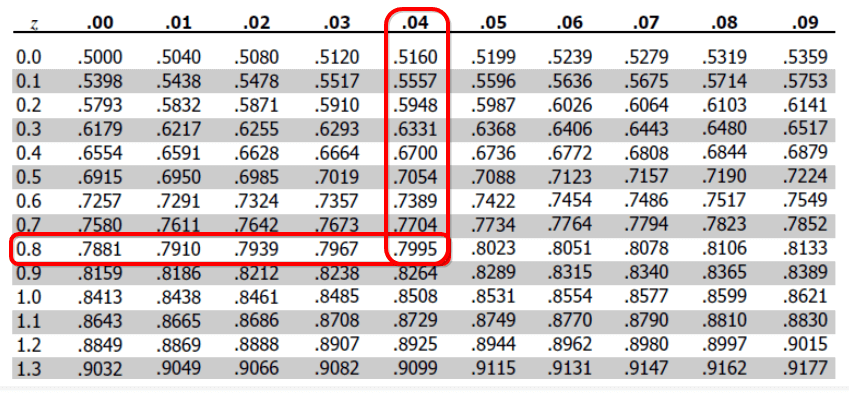Now, we must convert $$z$$ to $$x$$:

$z = \frac{x-\mu}{\sigma}$

$x =z\sigma + \mu = 0.84 \times 5+30=34.2$

The 80th percentile for the pizza delivery time in the city 'A' is 34.2 minutes.

If you use computer software, you can use the following commands:

Computer Software Package Command
Python  from scipy.stats import norm 
 norm.ppf(x, mu, sigma) 
MATLAB  norminv(p, mu, sigma) 
Excel  NORMINV(x, mu, sigma) 

from scipy.stats import norm

norm.ppf(0.8, 30, 5)
34.20810616786457


norminv(0.8, 30, 5)

34.2081


## Confidence interval

A normally distributed random variable is described by mean $$(\mu)$$ and standard deviation $$(\sigma)$$. A confidence interval is a probability that a parameter falls between a set of values for a certain proportion of times.

Assume a weight measurement of 80kg with a measurement standard deviation $$(\sigma)$$ of 2kg. The probability that the true weight falls between 78kg and 82kg is 68.25%.

Usually, we are interested in higher confidence levels, such as 90% or 95%. Let us see how to find it.

The following plot describes the standard normal distribution $$(\mu=0, \sigma=1)$$. We want to find a 90% confidence interval.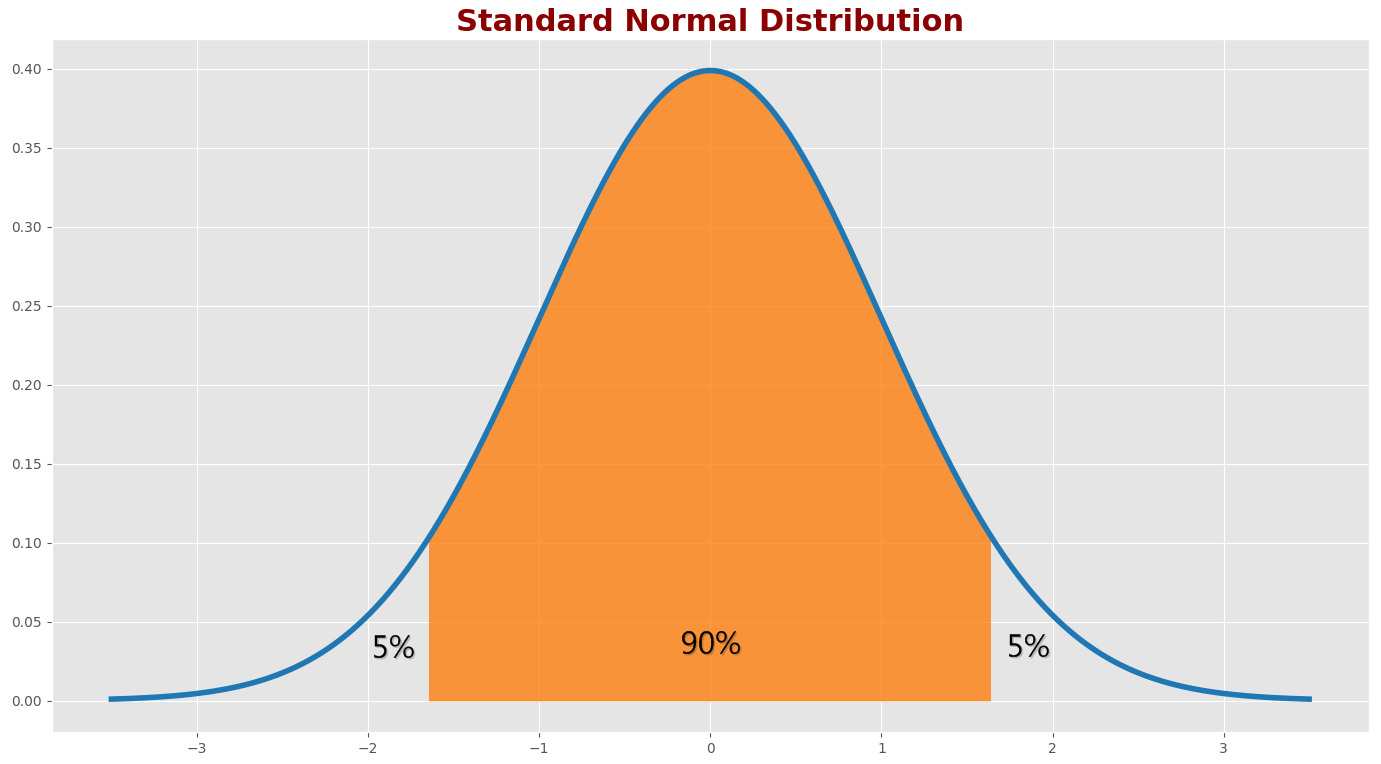The area of the filled region under the curve is 90% of the total area. The area of the unfilled region is 10% of the total area. The area of the unfilled region on the left is 5% of the total area. We can find a z-score for percentile 5 or percentile 95.


from scipy.stats import norm

norm.ppf(0.05)
-1.6448536269514729

norm.ppf(0.95)
1.6448536269514722



norminv(0.05)
-1.6449

norminv(0.95)
1.6449


The 90% confidence interval is $$(\pm 1.645 \sigma)$$.

For the weight measurement example, the 90% confidence interval is ±3.29kg. The probability that the true weight falls between 76.71kg and 83.29kg is 90%.